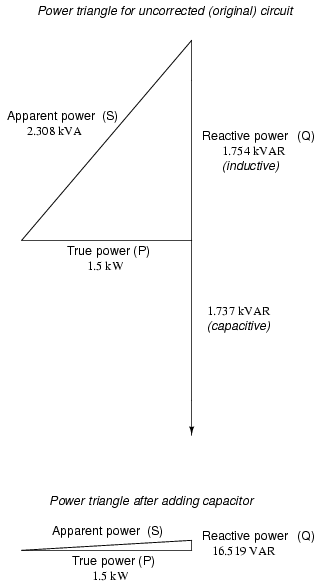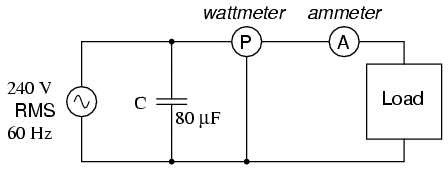# Why is capacitor placed in parallel for power factor correction?

I understand that to correct the power factor you have to choose a capacitance such that the reactive power from the circuit is cancelled by the reactive power of the circuit using this method.Circuit with power factor corrected:Why does one place the capacitor in parallel (as opposed to series)?

• One practical reason is that the capacitor would cause a voltage drop at the load. Another is that the capacitor would have to carry all the load current rather than just the reactive part. Apr 2, 2016 at 13:43
• Thanks. So would power factor correction still be achieved if the 80uF capacitor was placed in series but for those reasons you gave it is better to place it in parallel? edit: oh I think it's because in the calculations the voltage drop across the capacitor has to be 240Vrms Apr 2, 2016 at 13:49

Without the capacitor, the source has to provide all the energy (St): the actual energy consumed by the load (Pt) and the energy stored in the inductive part of the load (Qt). The inductive part makes the source supply a lot more current than necessary, since a lot of that current goes into setting up a magnetic field that stores some of the energy generated by the source.

With the capacitor in parallel, there is now an additional source of energy, which can take up some/all of the burden of supplying current to the inductive load (when it resists changes in current till it sets up its field), after which the source takes over again and recharges the capacitor. So the apparent power S (and thus energy) drawn from the source is reduced and is much closer to the true power P actually being used by the load.

Current can only flow in a closed loop, so a series capacitor cannot keep reactive current from flowing through the distribution grid, which is the very thing that power factor correction seeks to avoid in order to avoid the resistive losses of that current travelling long distances through practical conductors. Basically, the only way a series compensating capacitor could be effective for power factor would be to tune out the ability of the machine to draw power at line frequency at all, which would make it non-operational.

In contrast, parallel connection of an appropriately sized capacitor keeps the reactive current local, constrained to short low-loss wiring runs.

Let

• $$\R_C\$$ and $$\C\$$ be the resistance and capacitance of our capacitor, respectively
• $$\R_L\$$ and $$\L\$$ be the resistance and inductance of our load, respectively

Then

• $$\Z_C = R_C + \frac{1}{j\omega C}\$$ is the impedance of our capacitor
• $$\Z_L = R_L + j\omega L\$$ is the impedance of our load

As you know, the purpose of power factor correction is simply to decrease our apparent power usage, making it equal to (ideally) our real power usage.

# The problem with series capacitors

With a series capacitor, the voltage seen by our load would become $$V_L = V_S\left|\frac{Z_L}{Z_C + Z_L}\right|$$

But, to prevent overvoltage/undervoltage problems (among other things!), we must ensure that $$V_L = V_S \Longrightarrow \left|Z_C+Z_L\right| = \left|Z_L\right|$$

which, thereby, defeats the whole point of power factor correction altogether! That is, since our total impedance stays the same as before, we still end up drawing the exact same amount of apparent power as before! So, we win absolutely nothing with this approach to power factor correction.

# The benefit of parallel capacitors

With a parallel capacitor, our load always sees the full voltage $$\V_{S}\$$ anyway.

So, to correct the power factor, an ideal parallel capacitor will simply make $$\operatorname{Im}\left(\frac{Z_CZ_L}{Z_C+Z_L}\right) = 0 \Longrightarrow C=\frac{L}{\left|Z_L\right|^2}$$ for a new total impedance of $$\left|\frac{Z_CZ_L}{Z_C + Z_L}\right| = \frac{\left|Z_L\right|^2}{R_L} > \left|Z_L\right|$$ which means we'll draw less apparent power than before -- thus, satisfying the objectives of power factor correction!

## But, what about real capacitors?

Even though all real capacitors have some $$\R_C > 0\$$, the above calculations should be still be valid as long as $$\R_C \ll \left|Z_L\right|\$$.

But, even with high values of $$\R_C\$$, there is still value in doing power factor correction! The only difference is that now we're no longer seeking a power factor of 1 ("unity"), since now we also have to take into account the real power usage of our "real" capacitor as well.

So, for a capacitor with a large $$\R_C>0\$$, we would make $$C_{real} = \frac{\left|Z_L\right|\sqrt{\left|Z_L\right|^2+4R_C\left(R_C+R_L\right)}-\left|Z_L\right|^2-2R_CR_L}{2w^2R_C^2L}$$

The resulting total impedance will still be greater than our original $$\\left|Z_L\right|\$$, and, consequently, our apparent power usage still gets reduced!

Furthermore, note that $$\lim\limits_{R_C\to 0} C_{real} = C_{ideal} = \frac{L}{\left|Z_L\right|^2}$$ is also the same value as we had calculated for an ideal capacitor before.

if you put series you will create distorted output (limiting the current) which is why in cheaper led bulb the controller output is only Mylar Cap.

if you put parallel both L and N will surpresed against high amperage reactance power from the load. capacitor in AC parallel for PFC working like dampening the load. yes it's charging and giving output in the next cycle so your reactance power decreasing.

if you keep both series you will get current very limited by capability of your capacitor which in many cases will be blown out by overheat occurred by current flowing through it.

if you want to lower inrush current you can try NTC in series with line. it will giving you protection against high amperage starting.